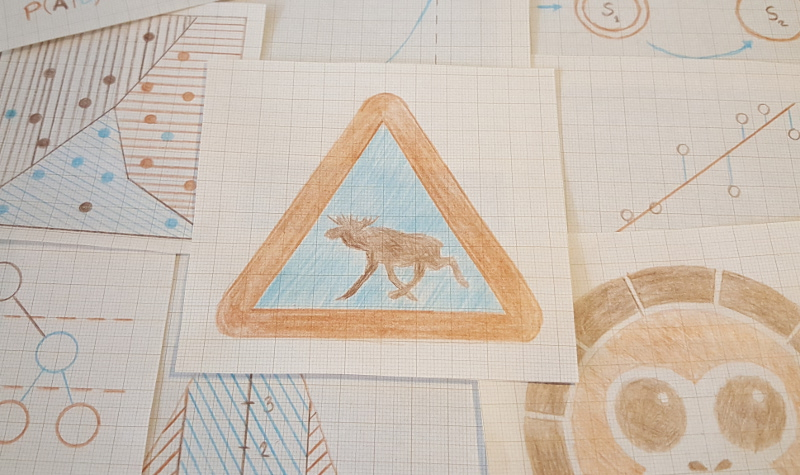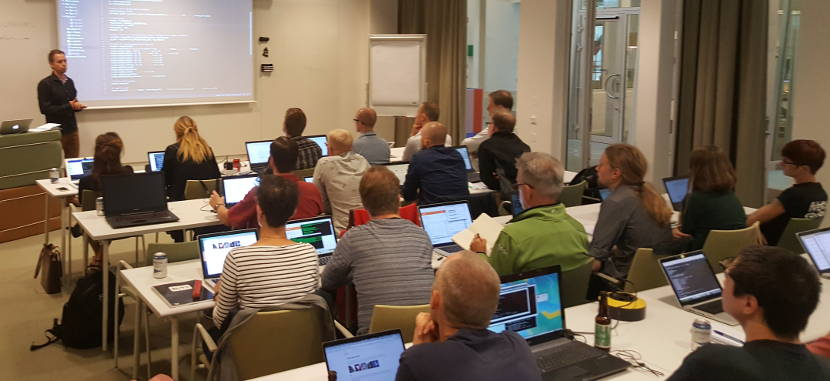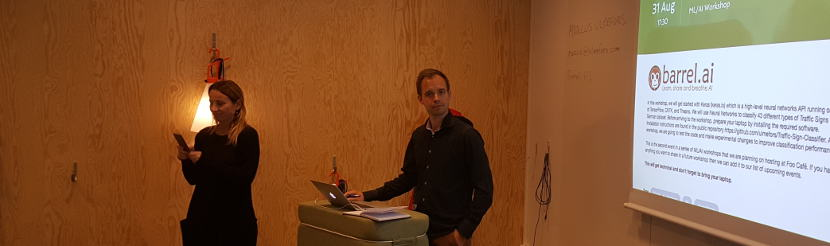# Image classification

25 AI monkeys showed up at Foo Cafe for our workshop. Marcus presented an image classification neural net that was built using Keras (keras.io) on top of TensorFlow. We played around with learning rate, batch size, epochs and sample fraction in the neural network, in order to find the optimal classification performance.Some was also discussing that this kind of neural net could be used to tackle other problems. Neural nets could more or less be used as a general purpose classifier. One thing that we lacked in this sample though was the ability to classify a single image after we trained the network. Even if you only feed the application with one image, it can only tell you how well it predicted the image and not what prediction it actually made. So below, in the lower part, is a way that you at least can see the predictions of the network, using the function model.predict().

``````# Print metrics of validation set
print('')
print('*** Training Complete ***')
print('Elapsed time: %.1f seconds' % training_time)
score = model.evaluate(X_val, y_val, verbose=0)
names = model.metrics_names
print('*** Metrics ***')
for i in range(len(score)):
print('%s: %.4f' % (names[i], score[i]))

# model.predict will return an array with the result of the predictions.
pred = model.predict(X_val)
for predicted_class in range(len(pred[sign])):
if (pred[sign][predicted_class] > 0.9):
print('%.2f percent sure that img %s is of class %s' % ((pred[sign][predicted_class]*100), sign, predicted_class))
``````

If you missed the event and want to play with the code, then the installation instructions can be found in the public repository https://github.com/ulmefors/Traffic-Sign-Classifier.

In order to see the tensorboard web page with the nice overview of the results during training. Type tensorboard --logdir=runs into your console and copy the provided link to your browser.

It was nice to meet so many people, eager to learn more about, AI. Hope to see you soon again!

// The Barrel Team- Image Classification, Neural Net, Keras, Tensorflow

Bayesian predictive inference machines >>